• ## 均匀分布随机数

千次阅读 2019-01-16 10:10:01
从均匀分布中进行采样，即生成均匀分布随机数，几乎是所有采样算法都需要用到的基本操作。然而，即使是如此简单的分布，其采样过程也并不是显然的，需要精心设计一定的策略。知识点概率统计，线性同余问题　如何编程...
场景描述
均匀分布是指整个样本空间中的每一个样本点对应的概率（密度）都是相等的。根据样本空间是否连续，又分为离散均匀分布和连续均匀分布。均匀分布可以算作是最简单的概率分布。从均匀分布中进行采样，即生成均匀分布随机数，几乎是所有采样算法都需要用到的基本操作。然而，即使是如此简单的分布，其采样过程也并不是显然的，需要精心设计一定的策略。
知识点
概率统计，线性同余
问题　如何编程实现均匀分布随机数生成器？
难度：★☆☆☆☆
分析与解答
首先需要明确的是，计算机程序都是确定性的，因此并不能产生真正意义上的完全均匀分布随机数，只能产生伪随机数（伪随机数是指这些数字虽然是通过确定性的程序产生的，但是它们能通过近似的随机性测试）。另外，由于计算机的存储和计算单元只能处理离散状态值，因此也不能产生连续均匀分布随机数，只能通过离散分布来逼近连续分布（用很大的离散空间来提供足够的精度）。
一般可采用线性同余法（Linear Congruential Generator）来生成离散均匀分布伪随机数，计算公式为（8.1）
也就是根据当前生成的随机数xt来进行适当变换，进而产生下一次的随机数xt+1。初始值x0称为随机种子。式（8.1）得到的是区间[0,m−1]上的随机整数，如果想要得到区间[0,1]上的连续均匀分布随机数，用xt除以m即可。
可以看出，线性同余法得到的随机数并不是相互独立的（下一次的随机数根据当前随机数来产生）。此外，根据式（8.1），该算法最多只能产生m个不同的随机数，实际上对于特定的种子，很多数无法取到，循环周期基本达不到m。如果进行多次操作，得到的随机数序列会进入循环周期。因此，一个好的线性同余随机数生成器，要让其循环周期尽可能接近m，这就需要精心选择合适的乘法因子a和模数m（需要利用代数和群理论）。具体实现中有多种不同的版本，例如gcc中采用 的glibc版本：但不管怎样，由计算机程序实现的随机数生成器产生的都是伪随机数，真正的随机数只会存在于自然界的物理现象中，比如放射性物质的衰变，温度、气流的随机扰动等。有一些网站可以提供基于大自然的随机现象的随机生成器，有兴趣的读者可以尝试一下。图8.1是通过大气噪声来产生随机数，可以说是“货真价实”的真随机数生成器了。面试时，面试官还可能会针对线性同余法进行深入提问，比如，线性同余法中的随机种子一般如何选定？如果需要产生高维样本或大量样本，线性同余法会存在什么问题？如何证明上述线性同余发生器得到的序列可以近似为均匀分布（伪随机数）？
来源：我是码农，转载请保留出处和链接！
本文链接：http://www.54manong.com/?id=1210

'); (window.slotbydup = window.slotbydup || []).push({ id: "u3646208", container: s }); })();

'); (window.slotbydup = window.slotbydup || []).push({ id: "u3646147", container: s }); })();
展开全文• 该方法不用产生高斯分布伪随机数，只需在均匀分布随机数的基础上设置不同的判决门限即可得到不同的误码率，误码率和误码的位置可控。硬件实验表明，该方法比采用高斯随机数的方案节省约96%的资源。
• 通过均匀分布随机数产生器获得一个高斯分布随机数产生器
一.Box-Muller Transfrom

对于给定一个能产生(0,1)之间的均匀分布的随机数产生器，可以利用Box-Muller transform来获得一个产生标准高斯分布随机数的随机数产生器。

Box-Muller transform可以描述如下：

假设U1和U2是两个独立的随机变量，并且其分布为(0,1)之间的均匀分布，可以得到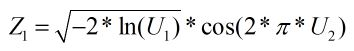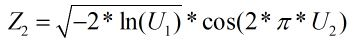Z1和Z2是两个互相独立的随机变量，并且这两个随机变量的分布为标准高斯分布。

二.Matlab实验

代码

%% clear

clc;

clear;

%% uniform distribution

N = 1000000;

U1 = rand(1, N);

U2 = rand(1, N);

%% Get Gussion distribution using box-Muller transfrom

Z1 = sqrt(-2 * log(U1)) .* cos(2 * pi * U2);

Z2 = sqrt(-2 * log(U1)) .* sin(2 * pi * U2);

%% visualisztion of PDF

subplot(2, 2, 1), hist(U1, 100), title( 'The PDF of U1' );

subplot(2, 2, 2), hist(U2, 100), title( 'The PDF of U2' );

subplot(2, 2, 3), hist(Z1, 100), title( 'The PDF of Z1' );

subplot(2, 2, 4), hist(Z2, 100), title( 'The PDF of Z2' );

U1,U2,Z1和Z2的概率密度如下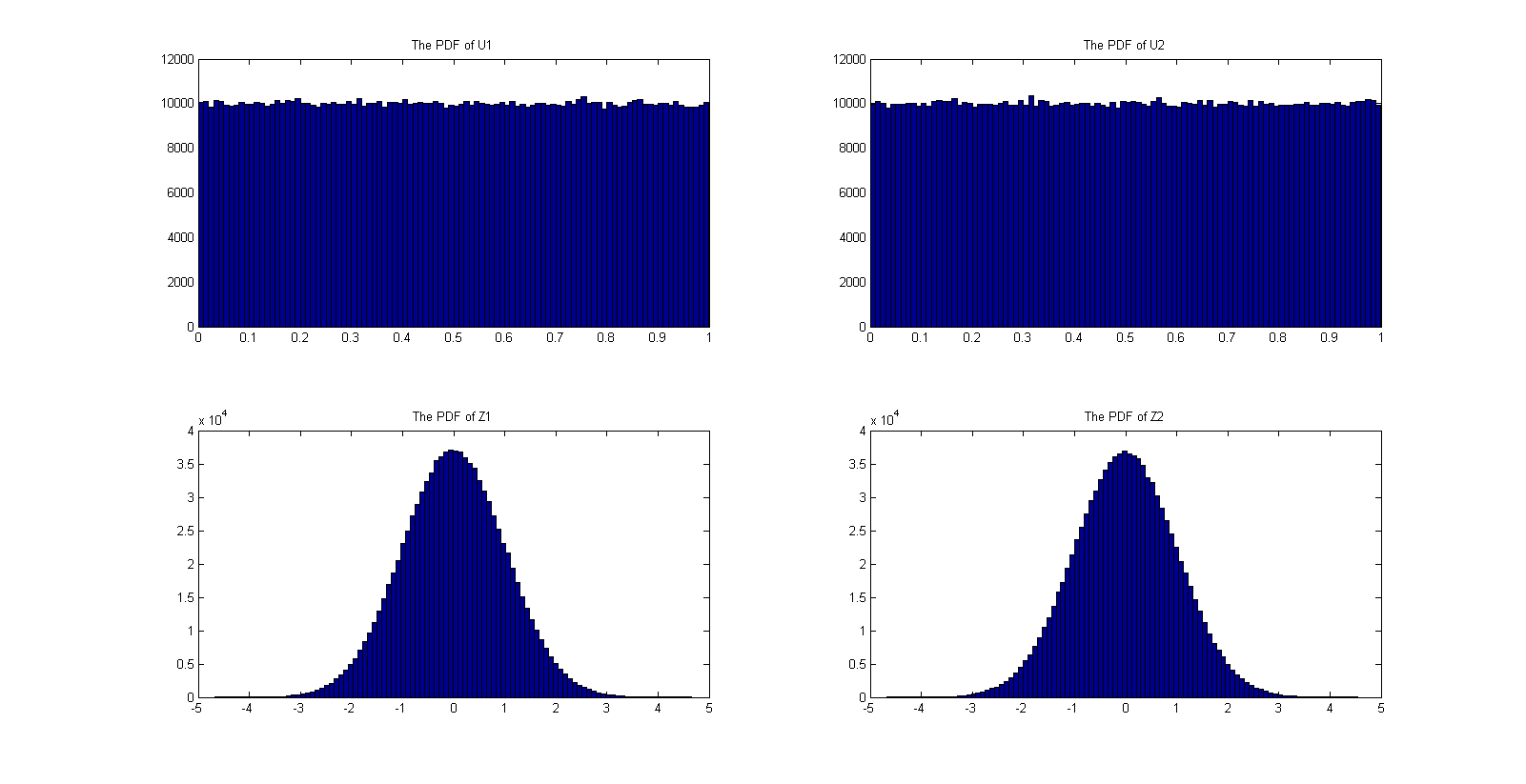参考文献

https://en.wikipedia.org/wiki/Box%E2%80%93Muller_transform

版权所有，欢迎转载，转载请注明出处，谢谢展开全文算法 高斯分布 Box-Muller
• Javascript 产生服从均匀分布随机数 大家都知道Math.random是 javascript 中返回伪随机数的方法，但Math.random()产生 [0,1）区间的随机数(注意，不包括1)，如果要取到1，可以这样：Math.floor(Math.random()*2);，...


文章目录
1 产生服从均匀分布随机数2 产生服从正态分布随机数3 产生服从柯西分布随机数

1 产生服从均匀分布随机数
大家都知道Math.random是 javascript 中返回伪随机数的方法，但Math.random()产生 [0,1）区间的随机数(注意，不包括1)，如果要取到1，可以这样：Math.floor(Math.random()*2);，取到 [0,2）之间的数然后向下取整，得到[0,1]。
取整数随机数要使用 floor 函数向下取整，而不能使用 round 函数四舍五入取整，round 取整后得到的是非均匀分布。
如果要取得特定范围的随机数可以对Math.random进行四则运算 例如：
//[10,20)
a=Math.random*10+10;
//[10,20]
a=Math.floor(Math.random()*11+10);

2 产生服从正态分布随机数
正态分布即高斯分布
Box-Muller变换是通过服从均匀分布的随机变量，来构建服从正态分布的随机变量的一种方法。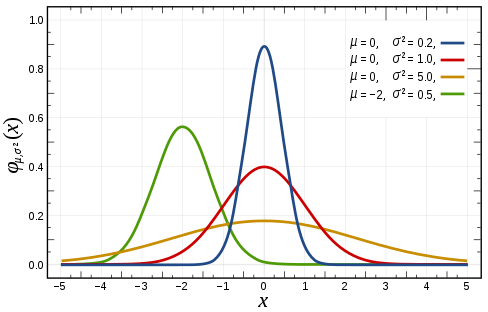function normalRandom(mean, std) {
let u = 0.0, v = 0.0, w = 0.0, c = 0.0;
do {
//获得两个（-1,1）的独立随机变量
u = Math.random() * 2 - 1.0;
v = Math.random() * 2 - 1.0;
w = u * u + v * v;
} while (w == 0.0 || w >= 1.0)
//Box-Muller转换
c = Math.sqrt((-2 * Math.log(w)) / w);
let normal = mean + (u * c) * std;
return normal;
}
//用于生产服从正态分布的随机数矩阵
function normalRandomSize(mean,std,size){
let normal = [];
for(let i=0;i<size;i++){
normal[i]=randomNormal(mean,std);
}
return normal;
}

3 产生服从柯西分布随机数
柯西分布的概率密度函数为

f

(

x

)

=

β

π

[

β

2

+

(

x

−

α

)

2

]

β

>

0

f(x)=\frac{\beta }{\pi [\beta ^{2}+ (x - \alpha)^{2}]} \qquad \beta > 0

通常用

C

(

α

,

β

)

C(\alpha ,\beta )

表示，其分布函数为

F

(

x

)

=

1

2

+

1

π

a

r

c

t

a

n

(

x

−

α

β

)

F(x)=\frac{1}{2}+\frac{1}{\pi }arctan(\frac{x-\alpha}{\beta })

用逆变换法产生柯西分布(Cauchy(\alpha ,\beta ))的随机变量(x)，产生均匀分布的随机数

u

u

，计算具体方法如下：

x

=

α

−

β

t

a

n

(

π

u

)

x=\alpha - \frac{\beta }{tan(\pi u)}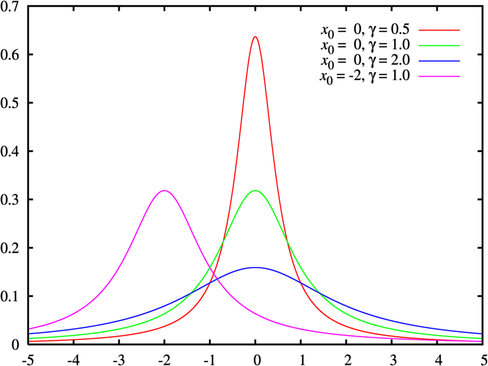function cauchyRandom(a,b) {
let u,cauchy;
u = Math.random();
cauchy = a - b / Math.tan(Math.PI * u);
return cauchy;
}


展开全文js
• pythonCSS布局HTML小编今天和大家分享正态分布的随机数Python编程产生非均匀随机数的几...从直观意义上理解，因为r一一对应着x,而在[0,1]均匀分布随机数R≤r的概率P(R≤r)=r。Python如何生成服从某个概率分布的随机...

pythonCSS布局HTML小编今天和大家分享正态分布的随机数
Python编程产生非均匀随机数的几种方法代码
如果函数y=f(x)是定义域D上的单调函数,那么f(x)一定有反函数存在,且反函数一定是单调的。分布函数F(x)为是一个单调递增函数，所以其反函数存在。从直观意义上理解，因为r一一对应着x,而在[0,1]均匀分布随机数R≤r的概率P(R≤r)=r。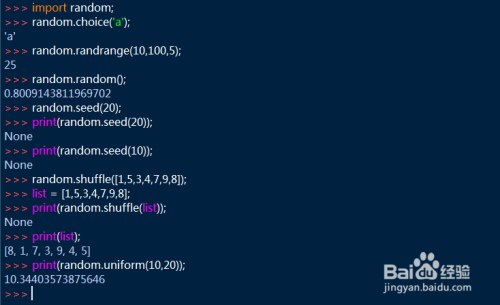Python如何生成服从某个概率分布的随机数
也可直接点“搜索资料”搜索整个问题。 概率分布 随机数 python 服从 搜索资料 本地图片 图片链接 代码 提交回答 匿名 回答自动保存中
python中用random.sample()生成的一组随机数，怎么例如 a=random.sample([1,2,3,4,5,6,7],3) 输入什么代码能让a从小到大排保存到列表里面。
python如何一次性取出多个随机数
关于Python中的随机数生成步骤和随机数质量
CSS布局HTML小编今天和大家分享大神讲解写Python2.7里面random随机数生成模块中随机数生成的详细步骤Python生成随机数和随机数质量的方法，random.random()用于生成一个指定范围内的随机符点数，两个参数其中一个是上限，一个是下限。如果a > b，则生成随机数： print random.uniform(10, 20) print random.uniform(20, 10) # #18.7356606526
如何用python编写一个产生1~100的随机数程序
需要用到random模块 import random n = random.randint(1,10) 会生成一个1到10的随机整数。 插入列表即可 [].append(n)
今天的python培训作业，CSS布局HTML小编今天和大家分享帮助，最好详细一点～
random模块 随机整数：random.randint(a,b)：返回随机整数x,a
如何用python生成两组随机数〈各50个，范围1

展开全文• 针对利用线性反馈移位寄存器生成均匀分布随机数序列连续值之间呈现出较强相关性的问题，研究了一种多位前跳技术，并采用该技术在可编程门阵列硬件中，高效紧凑地实现非相关、均匀分布随机数序列并行量化输出。...
• 有一个函数random()，该函数只能返回0或者1； 该函数以概率p返回1， 1-p的概率返回0.；   ...1. 一个函数randomUpdate(), ...2. randomUpdate（）可以任意调用random（）函数；...3.randomUpdate（）函数只能返
• 如何用均匀分布随机数生成正态分布随机变量 前言 在Monte Carlo模拟技术中，许多地方都需要用到符合标准正态分布(高斯)的随机数来设计采样方案，因此了解如何用均匀分布随机数(实际上是均匀分布的伪随机数)来...
• 生成均匀分布随机数的准备 准备随机数生成引擎，这里用到默认引擎——defalut_random_engin my_engine_name 创建均匀分布随机数生成器实例——uniform_int_distribution my_object_name(LOW, HIGH)（两边均为闭区间...C++均匀分布
• 使用均匀分布随机数产生器构造任意分布的随机数产生器
• 均匀分布随机数，线性同余法，反馈位寄存器法，组合随机数发生器线性同余法
• 本博文源于matlab基础，旨在讲述如何用matlab生成均匀分布随机数。一共有三种调用方式：matlab
• 混合同余法产生均匀分布随机数产生方法详细总结及其相应的改进方法。。。。。。混合同余法
• 均匀分布随机数       均匀分布是指在整个样本空间中的每一个样本点对应的概率（密度）都是相等的。根据样本空间是否连续，又分为离散均匀分布和连续均匀分布。均匀帆布可以算作是最...机器学习 人工智能
• 文章目录1. 生成随机数分布直方图2. 生成随机数矩阵，服从均匀分布 ...xlabel('[0,1]上均匀分布随机数'); % 为X轴加标签 ylabel('频数'); % 为Y轴加标签 2. 生成随机数矩阵，服从均匀分布 rand('twister',1); %matlab 直方图 矩阵
• 产生0-1之间一个均匀分布随机数 方法：参见《常用算法程序集》317页，徐士良。 传入参数:r--双精度实型变量指针,指向的单元存放随机数种子值//
• 乘同余法产生（0，1）均匀分布随机数的matlab代码，可在此基础上改进为不同分布的随机数，调试通过的
• 利用FPGA产生均匀分布随机数，原理简单，实现方便，消耗的资源较少。
• 均匀分布随机数 1.变换法 变换法主要分为两种：逆变换法和函数变换法 （1）逆变换法 逆变换法：如果我们希望生成服从的分布，那么可以通过U~U（0,1），Y=~F(x) （2）函数变换法 函数变换法分为一维与二维。 ...算法 统计学
• 从均匀分布中进行采样，即生成均匀分布随机数， 几乎是所有采样算法都需要用到的基本操作。然而，即使是如此简单的分布，其采样过程也并不是显然的，需要精心设计一定的策略。如何编程实现均匀分布随机数生成器？ ...
• np.random.rand用法 ...生成特定形状下[0,1)下的均匀分布随机数 np.random.rand(a1,a2,a3...)生成形状为(a1,a2,a3...),[0,1)之间的 均匀分布 随机数 np.random.rand(3,2) array([[ 0.14022471, 0.96360618], ...
• 随机数的意义 现实生活中，有很多场景需要用到“随机数”： 彩票 棋牌游戏中的洗牌和掷骰子 游戏掉宝率 其中大部分是靠计算机软件生成的的“伪随机数”。伪随机数一般是由随机种子和随机算法计算生成的，也就是...乘同余算法
• import tensorflow as tf f = tf.random.uniform([2,2],minval=0,maxval=1) print("f:",f) """ f: tf.Tensor( [[0.880005 0.91807914] [0.39310634 0.873947 ]], shape=(2, 2), dtype=float32) ...
• 随机数的使用非常广泛，例如在从统计总体中抽取有代表性的样本时，或者在将实验动物分配到不同的试验组的过程中，或者...二、转换变形方法，将均匀分布直接转换为高斯分布；三、拒绝采样，在转换方法中增加了按条件...c++生成随机数 c++随机数生成 c生成随机数
• 下面代码演示使用c++11的random库进行随机数的生成 #include &lt;iostream&gt; #include &lt;random&gt; using namespace std; int main() { std::mt19937 rng; rng.seed(std::random_device()...c
• 标准均匀分布是一个在范围 [0，1) 内的连续分布。generate_canonical() 函数模板会提供一个浮点值范围在 [0，1) 内，且有给定的随机比特数的标准均匀分布。它有 3 个模板参数：浮点类型、尾数的随机比特的个数，以及......